Volume 64 Supplement 10

# Applicability of CADMAS-SURF to evaluate detached breakwater effects on solitary tsunami wave reduction

## Abstract

Detached breakwaters, made with wave-dissipating concrete blocks such as Tetrapods, have been widely applied in Japan, but the effectiveness of such kinds of detached breakwaters on tsunami disaster prevention has never been discussed in detail. A numerical wave flume called CADMAS-SURF has been developed for advanced maritime structure design. CADMAS-SURF has been applied mainly to ordinary wave conditions such as wind waves, and little attempt has been made for expanding its application to tsunami waves. In this study, the applicability of CADMAS-SURF for evaluating the effectiveness of detached breakwaters on a solitary tsunami wave reduction is investigated by comparing the calculated results with those from hydraulic experiments. First, the effectiveness of a detached breakwater on the reduction of wave height and wave pressure was confirmed both by hydraulic experiments and numerical simulations. Finally, CADMAS-SURF has been found to be a useful tool for evaluating the effects of detached breakwaters on tsunami wave height and pressure reduction, as a first step in a challenging study.

## 1. Introduction

Coastal and port-related structures have been designed based on design formula as well as hydraulic model tests. Although hydraulic model tests can precisely reproduce actual physical phenomena, it usually requires time and cost to create seabed configurations and model structures, and to measure various kinds of data such as wave height, wave pressure, overtopped water and the movement of targeted structures. Also, the design formula is usually limited by the range of model conditions that the formula is based on. In addition, more information is required from the viewpoint of reliability-based performance design taking damage level into consideration.

Based on the above situation and recent advances in computer simulation technology, a numerical wave flume called CADMAS-SURF (e.g. Isobe et al., 1999) has been developed for advanced maritime structure design. CADMAS-SURF has been applied mainly to ordinary wave conditions such as wind waves, e.g. wave force onto breakwaters and wave overtopping of seawalls (e.g. Isobe et al., 2002; Goda and Matsumoto, 2003). So far, little attempt has been made to extend its application to tsunami waves.

Maritime structures are exposed to not only wind waves, but also tsunami waves. Damage to coastal structures such as seawalls were actually reported at the time of the South West Hokkaido earthquake tsunami in 1990, and the Japan Sea earthquake tsunami in 1983 (Tanimoto et al., 1983; Tanaka et al., 1993). Researchers have focused their efforts on the study of disaster prevention from tsunamis, especially with seawalls, e.g. Mizutani and Imamura (2000), Asakura et al. (2002) and Kato et al. (2006). In Japan, detached breakwaters have been widely applied, but the effectiveness of detached breakwaters on tsunami disaster prevention has never been discussed in detail. At the time of the Indian Ocean Tsunami in December, 2004, in Male, the main island of the Maldives, detached breakwaters effectively protected the island from the tsunami (Fujima et al., 2006). However, the effectiveness of detached breakwaters on tsunami reduction has not been discussed in detail.

In recent years, the risk of the occurrence of tsunamis generated by near the shore large earthquakes, such as Tokai, Tonankai, Nankai and off-Miyagi earthquakes, is considered to be high. In addition, the occurrence of the great Chilean earthquake in February 2010 caused a large trans-Pacific tsunami. In this study, the applicability of the numerical simulation model called CADMAS-SURF for evaluating the effects of detached breakwaters on a solitary tsunami wave reduction will be discussed.

## 2. Numerical Wave Flume

### 2.1 Basic equations

The numerical wave flume in this study is called CADMAS-SURF (SUper Roller Flume for Computer Aided Design of MAritime Structure), and it is based on the following equations of continuity for a 2-dimensional non-compressive fluid and the Navier-Stokes formula: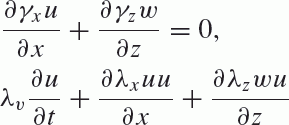((1))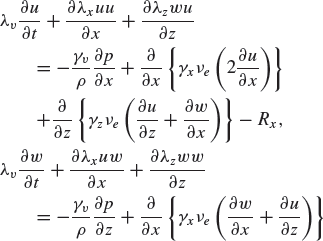((2))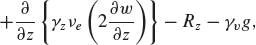((3))

where t is the time, x, z are the horizontal and vertical coordinates, respectively, u, w are the horizontal and vertical velocities, respectively, ν e is the molecular kinematic viscosity, γ v is the porosity, γ x , γ z are the horizontal and vertical sectional transform ratios, respectively, p is the pressure, ρ is the mass density of the fluid, and g is the acceleration due to gravity.

The coefficients of λ υ , λ x , λ z are: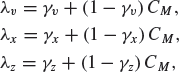((4))

where C M is the coefficient of inertia.

The horizontal and vertical drag forces R x , R z : follows.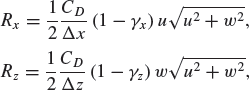((5))

where C D is the drag coefficient, and Δx, Δz are the horizontal and vertical mesh sizes for numerical simulations, respectively.

### 2.2 Free surface

In order to handle the free surface of the fluid, the VOF (Volume of Fluid) method (Hirt and Nichols, 1981) is applied. The transfer diffusion equation F of the VOF function is: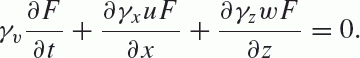((6))

## 3. Hydraulic Model Tests

### 3.1 Method of wave generation

In this study, a solitary wave, described by the following equations, is generated: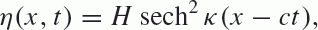((7))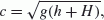((8))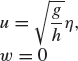((9))

with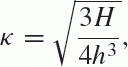((10))

where H is the wave height, c is the wave celerity, and h is the water depth.

When generating the solitary wave expressed as Eq. (7), the required wave paddle stroke S is given by Eq. (11), following Goring and Raichlen (1980).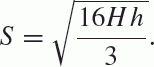((11))

The position of the wave making paddle X at time t is given as follows: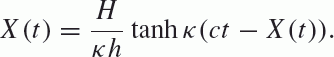((12))

Equation (12) cannot be solved analytically because of the inclusion of an unknown variable X (t) in the right-hand part and the non-linearity of X(t). Therefore, the wave paddle position Xi+1 at t = i + 1 is calculated based on Eq. (13), using X i at t = i by the Newton-Raphson method: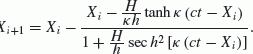((13))

The extreme situation at the time that gives the value of − 1 for tanh κ(ctX(t)) of Eq. (12) should be considered, because the wave length of the solitary wave is theoretically infinity. In this study, the time t0 giving the value −0.999 to tanh κ(ctX (t)) is obtained by Eq. (14) following Goring and Raichlen (1980). From this, the following wave paddle position X i , at each time t i , can be calculated by Eq. (13) with the initial time t0.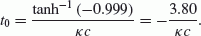((14))

### 3.2 Sea bed and structures

Figure 1 shows the wave flume set-up. The piston type wave maker is installed at x = 0 m. The slope of 1/5 begins at x = 3.75 m and ends at x = 4.25 m. The slope of 1/30 begins at x = 4.25 m and ends at x = 13.25 m. The flat bed is constructed from x = 13.25 m to 14.75 m followed by a 1/20 slope. This topography represents the typical cross-section around Japanese coasts.

In total, 13 wave gauges were installed from x = 2.25 m to 14.25 m (St. 1 to 13) for water surface monitoring as shown in Fig. 1.

Figure 2 shows the cross-section of the detached breakwater constructed in the flume. The center of the detached breakwater is set at x = 11.25 m (St. 9) as shown in Figs. 1 and 2. The detached breakwater is made using wave-dissipating concrete blocks of Tetrapods of 59 g with a porosity of 50%. The crown width of the detached breakwater is equivalent to 3 rows of Tetrapod units. The crown height is set with a clearance of 4 cm above the sea-water level which is 0.5 times the wave height equivalent to the stability limit of Tetrapods of 59 g based on ordinary design against wind waves. This is the common method for detached breakwater design in Japan.

The seawall was constructed at x = 13.75 m (St. 12), and 7 wave pressure gauges with a capacity of 1.96 N/cm2 were installed on the surface of the seawall as shown in Fig. 3.

Figure 4 is a photograph showing the detached breakwater, seawall and wave gauges, Fig. 5 is a photograph focusing on the cross-section of the detached breakwater.

Table 1 shows the hydraulic model test cases. Case 1 is the test case with no structures for checking the incident wave by measuring water surface change with wave gauges. Case 2 is the test case with a detached breakwater, and without a seawall, to analyze the detached breakwater effect on solitary-wave-height reduction. Cases 3 and 4 are the test cases with a seawall. Case 3 is the test case without a detached breakwater. Case 4 is the test case with a detached breakwater to analyze the effect of the detached breakwater on the reduction of wave pressure on the seawall. Suffix-1 and suffix-2 correspond to the high-water levels with an offshore water depth of 0.43 m (high-tide case) and 0.40 m (low-tide case), respectively. The wave height at St. 1 is set as H0 = 5.3 cm.

## 4. Numerical Simulations

As described before, in the hydraulic experiments, solitary tsunami waves were generated based on Eqs. (7) to (14). In the simulation in the numerical wave flume, the same method of wave generation was applied, i.e., the water level and velocity at each time obtained by Eqs. (7) and (9) were given at the wave generation boundary, x = 0 m, with the initial time of t0 as given by Eq. (14). Behind the wave maker, a wave damping area, called the sponge layer, of 4 m from x = −5 m to −1 m, was added to suppress wave reflection from the offshore end of the flume.

In the numerical simulations, the horizontal and vertical mesh sizes were set as Δx = 1.0 cm and Δz = 1.0 cm, respectively. Referring to previous researches, the appropriate horizontal mesh size should be chosen by satisfying the equation, L/ Δx > 80, where L is a wave length. In this study, the wave length L, corresponding to the time t0 obtained by Eq. (14), was 10.85 m for high tide, and 9.74 m for low tide, to satisfy the above criteria. On the other hand, the vertical mesh size was recommended to satisfy equations, Hz > 10 for general wave conditions, and Hz > 5 for the weak-linear wave with a wave height smaller than a breaking wave. In this study, H0 = 5.3 cm satisfies the condition Hz > 5.

The time interval Δt in the simulations is automatically calculated as Eq. (16), where Δt c , determined based on the following CFL condition of Eq. (15), is multiplied by a safety factor α. In this study, α is set as 0.2 based on a preliminary calculation.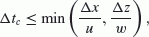((15))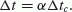((16))

The porosity γ v of the detached breakwater is 50% as mentioned before. The coefficients of drag force and inertia are set as C D = 1.0 and C M = 1.2, respectively, by following Sakakiyama and Imai (1996).

The wave flume set-up, as shown in Figs. 1 to 3, is also used in the numerical simulations, where water surface and wave pressure are calculated for the cases shown in Table 1.

## 5. Results and Discussions

### 5.1 Water surface

#### (1) Case 1-1

Figure 6 shows the time series of water surface variation without a detached breakwater at certain chosen locations. In this case, the offshore water depth is 43 cm, and that on the shore side is 3 cm. The initial offshore wave height at St. 1 is H0 = 5.3 cm. Figures 6(a) and (b) show the hydraulic experimental results and the simulated results, respectively. The wave deformation phenomena from the shoaling process up to St. 10 (x = 12.25 m) is successfully simulated by the numerical wave flume. Even though around St. 12 (x = 13.75 m) within the flat area the simulated result is biugger than that in the hydraulic experiment, the overall shape of the simulated wave agrees well with that of the hydraulic experimental wave.

#### (2) Case 1-2

The offshore water depth in Case 1-2 is 40 cm, which is shallower than that in Case 1-1 by 3 cm, and the water depth at the flat area is 0 cm. Figure 7 shows a time series of water surface variation similar to Fig. 6. Because the water depth is shallower than that in Case 1-1, the wave breaking point has moved offshore and the wave has broken before reaching St. 10 (x = 12.25 m). The numerical simulation results agree well with the hydraulic experimental results, but the wave shape landward from the wave breaking point shows less agreement.

#### (3) Case 2-1

Figure 8 shows the time series of the water surface variation for Case 2-1. Before the wave reaches the detached breakwater, the water surface variation is similar to that for Case 1-1. After the wave passes the breakwater wave reduction can be seen and is well simulated by the numerical wave flume. The wave reflection from the breakwater in the deeper region is also well simulated.

#### (4) Case 2-2

Figure 9 shows the time series of the water surface variation for Case 2-2. Similar to Case 2-1, the simulated results agree well with the experimental results both before and after the wave reaches the detached breakwater, as well as the reflected wave from the breakwater in the deeper region.

As shown in Figs. 6 to 9, the time series of the water surface can be well simulated by CADMAS-SURF before wave breaking. Some discrepancies between the simulated results and the experimental results of the detailed shape of the time series, after wave breaking, can be seen. This might be caused by the difficulty of simulating air bubble inclusion, due to wave breaking, in the numerical simulation.

In the numerical simulation, the water surface at later times tends to descend to a level lower than the initial sea-water level compared with the experimental results. The reason for these discrepancies has not yet been explained and will be considered in future work.

Although there are still problems to be solved, CADMAS-SURF merits application in maritime structure design against solitary tsunami waves with regard to tsunami disaster mitigation, because the incident mode of such waves is generally of critical relevance.

### 5.2 Wave height

As discussed before, wave height plays an important role regarding the stability of concrete blocks, and run-up and wave pressure on seawalls, from the perspective of tsunami disaster mitigation. Figures 10 and 11 show a comparison of simulated wave heights with experimental ones in Case 1 (without a detached breakwater) and Case 2 (with a detached breakwater) at all points of measurement in the hydraulic experiments, respectively. Without any structures, the results of the numerical simulation coincide with experimental data from deeper areas to wave-breaking points, for both high and low tide cases. At the locations landward from wave-breaking points, the simulated wave heights are smaller than the experimental ones by only 10% for the low-tide case (Case 1-2). On the other hand, for the high-tide case (Case 1-1), the differences are rather large. There might be a problem in simulating air bubble inclusion, due to wave breaking, in the numerical simulation as previously mentioned.

Figure 11 shows the results with a detached breakwater (Case 2). The numerical simulation can well reproduce the experimental data from the deep area to the position of a detached breakwater. At the positions landward from the detached breakwater, the simulated results are rather large compared with the experimental data in general and the difference becomes larger for a shallower area. This tendency can be explained by the smaller wave heights at the positions landward from the detached breakwater and the accuracy of the numerical simulation, which is lower in the shallower area. In the area where the wave shape becomes rather sharp around the breaking point, and where the wave energy is dissipated due to wave breaking, the vertical mesh size criteria cannot be satisfied. This can be one reason for the discrepancies noted above. In our study, a vertical mesh size of Δz = 1.0 cm was chosen by taking the computing time into account. The use of a smaller vertical mesh size to improve the accuracy of the numerical simulation is left for future research.

### 5.3 Wave pressure on the seawall

#### (1) Time series of wave pressure

Figure 12 shows the time series of the wave pressure of Case 3-1 for a high-tide case at the position of the S.W.L. where the wave pressure becomes a maximum among all the points of the wave-pressure gauges. The peak value of wave pressure by numerical simulation is a little different from the one obtained in the hydraulic experiment. However, the numerical simulation data shows good agreement with the experimental data for the second peak value and the overall shape of the time series.

Figure 13 shows the time series of the wave pressure of Case 3-2 for a low-tide case at the position of the S.W.L. where the wave pressure becomes a maximum similar to Case 3-1. In this case, the peak value of the wave pressure by numerical simulation agrees well with that in the hydraulic experiment, as well as in the total shape of the time series.

#### (2) Wave pressure distribution

As discussed in the section concerning water surface comparison, the maximum wave pressure should be taken into account with regard to the design of seawalls against tsunamis.

Figure 14 shows a comparison of the wave pressure distributions on the seawall for Case 3, without a detached breakwater, by numerical simulations and hydraulic experiment. In the hydraulic experiment, the waves are generated twice and both sets of data are plotted. The value pmax at each elevation is defined as the maximum wave pressure of the time series for each point. Therefore, the time of each pmax is not necessarily the same.

The overall shape of the wave pressure distribution for the high-tide case (Fig. 14(a)) by numerical simulation shows a fairly good agreement with the experimental results. However, the position of the maximum pressure is a little different. As for the low-tide case (Fig. 14(b)), the overall shape of the wave pressure distribution by numerical simulation also agrees well with the experimental results. Better agreement can be seen in the lower area than the upper area.

Figure 15 shows a similar comparison for Case 4 with a detached breakwater in the same manner as Fig. 14. In the high-tide case (Fig. 15(a)), the wave pressure peaks at just above the S.W.L. in the hydraulic experiment. On the other hand, in the numerical simulation, it peaks at the lowest position. However, good agreement is seen in the area under the S.W.L. In the low-tide case (Fig. 15(b)), the wave pressure in the numerical simulation at the higher position is lower that in the experiment. The wave pressure at the lowest position is almost the same in both tide cases.

#### (3) Effect of detached breakwater

Figure 16 shows a comparison of the wave pressure distributions with, and without, a detached breakwater. The clear wave pressure reduction by a detached breakwater can be seen in the figures. The wave pressure can be reduced to 60–70% by the detached breakwater. The highest position is also lowered. Therefore the total horizontal wave force is considered to be reduced to 40–45% by the detached breakwater.

As shown in Figs. 14, 15 and 16, the wave pressure distribution can be well simulated by CADMAS-SURF; however, some discrepancies can be noted. These might be caused by difficulties of simulating air bubble inclusion due to wave breaking and wave collision at the seawall in the numerical simulation, as discussed in Section 5.1. Improvement of the numerical simulation to address this discrepancy will be considered in future work.

## 6. Conclusions

The applicability of the numerical simulation called CADMAS-SURF for a solitary tsunami wave has been studied to evaluate the effects of detached breakwaters on wave pressure reduction at seawalls. The results of this study are summarized as follows:

1. (1)

Water surface variation before the wave reaches the wave breaking point, and up to the front of a detached breakwater was well simulated by the numerical simulations.

2. (2)

Wave pressure on a seawall was also well simulated.

3. (3)

The effectiveness of a detached breakwater on the reduction of wave height and wave pressure was confirmed both by hydraulic experiment and numerical simulations.

4. (4)

The applicability of CADMAS-SURF for tsunami disaster mitigation has been validated.

## References

• Asakura, R., K. Iwase, T. Ikeya, M. Takao, T. Kaneto, N. Fujii, and M. Ohmori, The tsunami wave force acting on land structures, Proc. 28th Int. Conf. Coast. Eng., ASCE, 1191–1202, 2002.

• Fujima, K., Y. Shigihara, T. Tomita, K. Honda, H. Nobuoka, M. Hanzawa, H. Fujii, H. Ohtani, S. Orishimo, M. Tatsumi, and S. Koshimura, Survey results of Indian Ocean Tsunami in the Maldives, Coast. Eng. J., JSCE, 48(2), 81–97, 2006.

• Goda, Y. and A. Matsumoto, A simplified theory on total wave pressure exerted on a rubble mound breakwater, Proc. Asia Pac. Coast., 2003.

• Goring, D. and F. Raichlen, The generation of long waves in the laboratory, Proc. 17th Int. Conf. Coast. Eng., 763–783, ASCE, 1980.

• Hirt, C. and B. D. Nichols, Volume of fluid (VOF) method for dynamics of boundaries, J. Comput. Phys., 39, 201–225, 1981.

• Isobe, M., Y. Xiping, K. Umemura, and S. Takahashi, Study on development of numerical wave flume, Proc. Coast. Eng., JSCE, 46, 36–40, 1999 (in Japanese).

• Isobe, M., Y. Hanahara, Y. Xiping, and S. Takahashi, Numerical simulation of waves overtopping a breakwater, Proc. 28th Int. Conf. Coast. Eng., ASCE, 2273–2285, 2002.

• Kato, F., S. Inagaki, and M. Fukuhama, Wave force on coastal dike due to tsunami, Proc. 30th Int. Conf. Coast. Eng., ASCE, 5150–5161, 2006.

• Mizutani, M. and F. Imamura, Hydraulic experimental study on wave force of a bore acting on structure, Proc. Coast. Eng., JSCE, 47, 946–950, 2000 (in Japanese).

• Sakakiyama, T. and S. Imai, Numerical simulation of wave overtopping at seawall covered by wave-dissipating concrete blocks, Proc. Coast. Eng., JSCE, 43, 696–700, 1996 (in Japanese).

• Tanaka, S., H. Ito, and K. Yamamoto, Disaster by Hokkaido Nanseioki Earthquake Tsunami, Kaigan, 33(2), 12–17, 1993 (in Japanese).

• Tanimoto, K., T. Takayama, K. Murakami, S. Murata, H. Tsuruya, S. Takahashi, M. Morikawa, Y. Yoshimoto, S. Nakano, and T. Hiraishi, Field and laboratory investigation of the tsunami caused by 1983 Nihonkai Chubu Earthquake, Technical Note of the Port and Harbour Research Institute Ministry of Transport, No. 470, 299 pp., 1983 (in Japanese).

## Author information

Authors

### Corresponding author

Correspondence to Minoru Hanzawa.

## Rights and permissions

The images or other third party material in this article are included in the article’s Creative Commons licence, unless indicated otherwise in a credit line to the material. If material is not included in the article’s Creative Commons licence and your intended use is not permitted by statutory regulation or exceeds the permitted use, you will need to obtain permission directly from the copyright holder.

To view a copy of this licence, visit https://creativecommons.org/licenses/by/4.0/.

Reprints and Permissions

Hanzawa, M., Matsumoto, A. & Tanaka, H. Applicability of CADMAS-SURF to evaluate detached breakwater effects on solitary tsunami wave reduction. Earth Planet Sp 64, 13 (2012). https://doi.org/10.5047/eps.2011.06.030

• Revised:

• Accepted:

• Published:

• DOI: https://doi.org/10.5047/eps.2011.06.030

### Key words

• Tsunami
• solitary wave
• detached breakwater
• seawall
• numerical simulation
• hydraulic model test